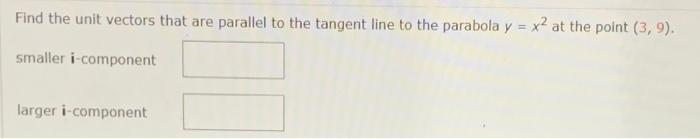# Question Solved1 AnswerFind smaller i component and larger i component Find the unit vectors that are parallel to the tangent line to the parabola y = x2 at the point (3,9). smaller i-component larger i-componentFind smaller i component and larger i componentTranscribed Image Text: Find the unit vectors that are parallel to the tangent line to the parabola y = x2 at the point (3,9). smaller i-component larger i-component
More
Transcribed Image Text: Find the unit vectors that are parallel to the tangent line to the parabola y = x2 at the point (3,9). smaller i-component larger i-component